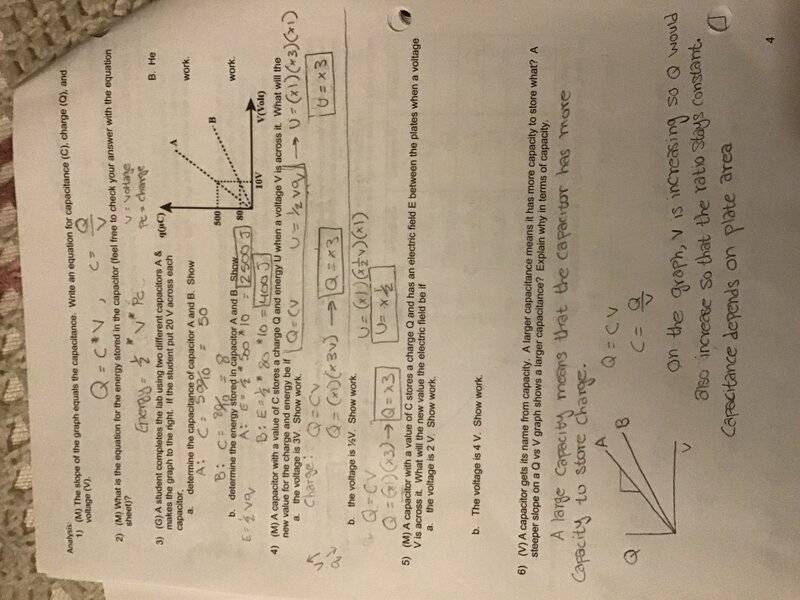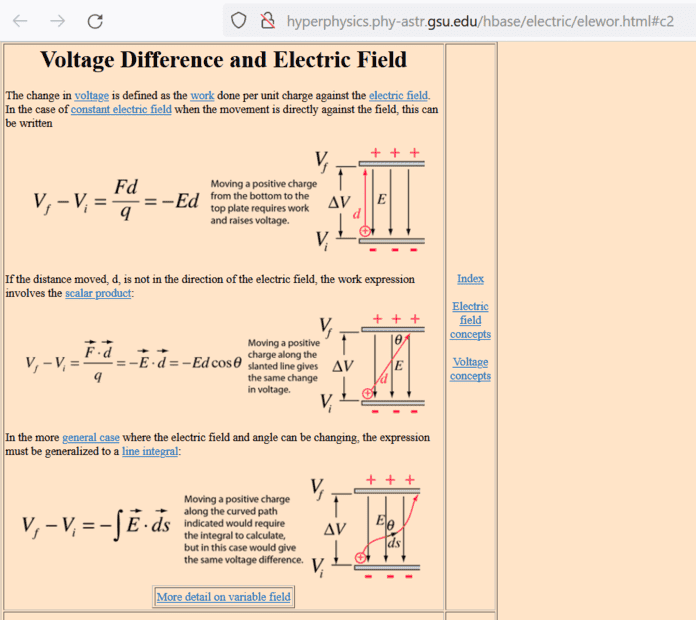# How do I incorporate electric fields into capacitors?

MinaciousOviraptor
Homework Statement:
A capacitor with a value of C stores a charge Q and has an electric field E between the plates when a voltage V is across it. What will the new value of the electric field be if:

a) the voltage is 2V
b) the voltage is 4V
Relevant Equations:
U=(1/2)CV^2
U=(1/2)QV
U= (1/2)Q^2V
Q=CV
I know that I’m supposed to use proportional reasoning, but where does electric field even fit in? For whatever equation, I know I’m supposed to see how increasing the voltage by either 2 and 4 volts related to electric field. If electric field is the same as “U”, then wouldn’t it be U=(1/2)*(1)*(3V) using the equation U=(1/2)QV?

Mentor
How does electric field relate to voltage? (That's all you need.)

MinaciousOviraptor
How does electric field relate to voltage? (That's all you need.)
But what’s the relevant equation? Is E-field the same as U?

Mentor
Is E-field the same as U?

YanZhen
Homework Statement:: A capacitor with a value of C stores a charge Q and has an electric field E between the plates when a voltage V is across it. What will the new value of the electric field be if:

a) the voltage is 2V
b) the voltage is 4V
Relevant Equations:: U=(1/2)CV^2
U=(1/2)QV
U= (1/2)Q^2V
Q=CV

I know that I’m supposed to use proportional reasoning, but where does electric field even fit in? For whatever equation, I know I’m supposed to see how increasing the voltage by either 2 and 4 volts related to electric field. If electric field is the same as “U”, then wouldn’t it be U=(1/2)*(1)*(3V) using the equation U=(1/2)QV?
Do you have original question?
Why U=(1/2)QV and U=(1/2)Q^2V?
(1/2)QV≠(1/2)Q^2V.I can't understand it.
Could you explain it?

MinaciousOviraptor
Do you have original question?
Why U=(1/2)QV and U=(1/2)Q^2V?
(1/2)QV≠(1/2)Q^2V.I can't understand it.
Could you explain it?It’s number 5- but that’s literally all it says. I don’t see a relationship to prior problems

YanZhen
E=U/d
d is a constant
so Ea=2E Eb=4E
and U is like mgh,E is like g.one in the electric field,one in the force field.
understand?

Mentor
Relevant Equations:: U=(1/2)CV^2
U=(1/2)QV
U= (1/2)Q^2V
Q=CV
The highlighted equation is incorrect.

As I stated before, you won't need any of these. Just the relationship between V and E. (Read the link I gave.)

YanZhen
C=Q/V C is a constant
E=F/q=((kQq)/(d^2))/q=kQ/(d^2)=kCV/(d^2)
is this the answer you want?

Homework Helper
Gold Member
C=Q/V C is a constant
E=F/q=((kQq)/(d^2))/q=kQ/(d^2)=kCV/(d^2)
is this the answer you want?
This is misleading and wrong. You are misleading the OP by asserting that the total charges ##\pm Q##, which are distributed uniformly over the plates, are point charges. It is wrong because the field due to two point charges is the superposition of two ##1/r## fields while the field between the capacitor plates is uniform and does not depend on ##r##.

YanZhen
This is misleading and wrong. You are misleading the OP by asserting that the total charges ##\pm Q##, which are distributed uniformly over the plates, are point charges. It is wrong because the field due to two point charges is the superposition of two ##1/r## fields while the field between the capacitor plates is uniform and does not depend on ##r##.
so,how should we describe the relationship between V and E?
i'm so out of ideas.

Mentor
so,how should we describe the relationship between V and E?

•MinaciousOviraptor
YanZhen
emmm.i can't open it.
could you send me the content?thank youMentor
emmm.i can't open it.
could you send me the content?thank youYou really can't open the Hyperphysics link? I wonder if it's some country blocking thing for your ISP. Here is a snapshot:Homework Helper
Gold Member
I wonder if it's some country blocking thing for your ISP.
Probably the Great Firewall. OP has self-identified as being in China.

•berkeman
YanZhen
Probably the Great Firewall. OP has self-identified as being in China.
yes

MinaciousOviraptor
So I’m disregarding Capacitance and Charge and just focusing on the relationship between V and E—> V=ED. If V=2V then E would also be 2

Mentor
So I’m disregarding Capacitance and Charge and just focusing on the relationship between V and E—> V=ED. If V=2V then E would also be 2
Yes, it's that simple. Doubling the voltage doubles the electric field -- they are proportional.

•MinaciousOviraptor
MinaciousOviraptor
Yes, it's that simple. Doubling the voltage doubles the electric field -- they are proportional.
Thank you!

•Doc Al and berkeman